What does it do?

Converts a formula to text and lets you specify the display formatting by using special format strings

Formula breakdown:

=TEXT(value1 – value2, format text)

What it means:

=TEXT(formula, a text string enclosed in quotation marks)

When you have two points in time and you want to calculate the amount of time elapsed, then you will need to use Excel´s TEXT function

Sometimes data gets dumped into Excel with the following date & time format:

24/01/2015  19:48:00.

Using the TEXT function and entering a special text string can give you the time elapsed in Days, Hours, Minutes and Hours & Minutes.

See below how easy this is to implement.STEP 1: Enter the following to get the elapsed time in days:

We need to enter the TEXT function in a blank cell:

## =TEXT(B12-A12, “dd”)

value1 is the end date time
value2 is the start date time
format text is “dd” which signifies days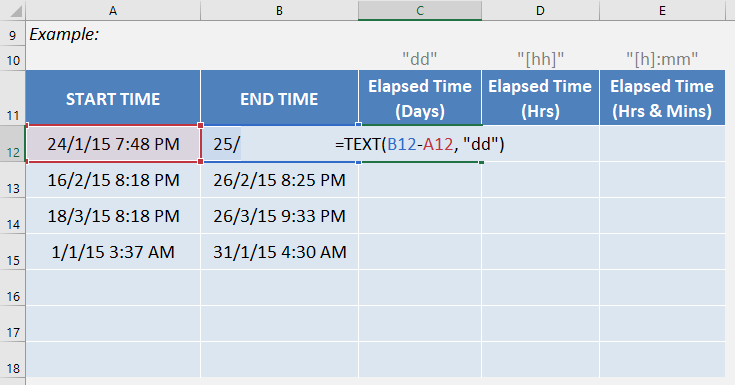STEP 2: Apply the same formula to the rest of the cells by dragging the lower right corner downwards.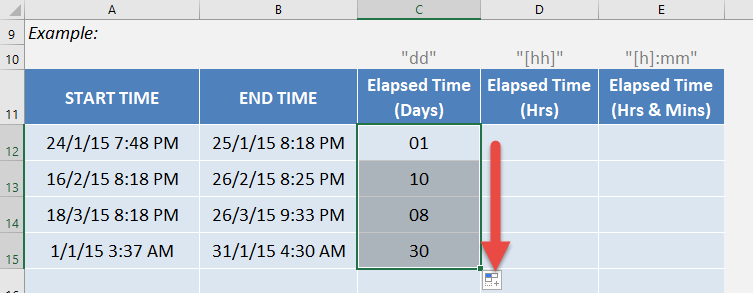STEP 3: Enter the following to get the elapsed time in hours:

We need to enter the TEXT function in a blank cell:

## =TEXT(B12-A12, “[hh]”)

value1 is the end date time
value2 is the start date time
format text is “[hh]” which signifies hours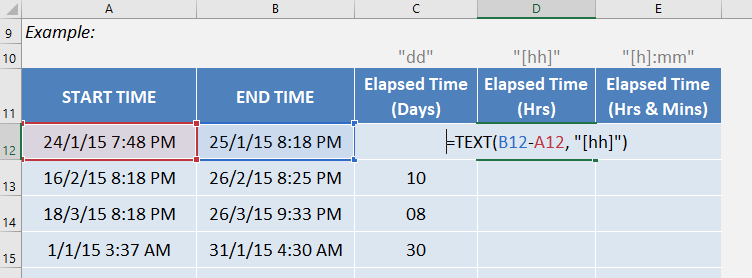STEP 4: Apply the same formula to the rest of the cells by dragging the lower right corner downwards.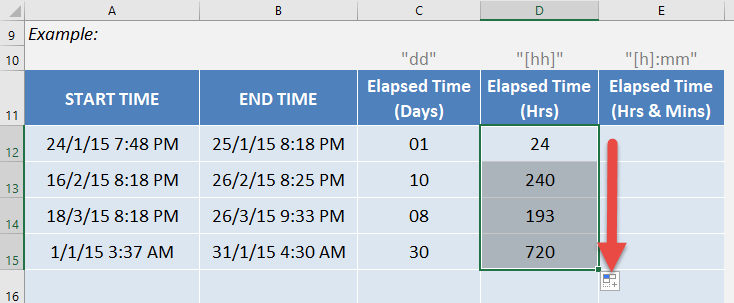STEP 5: Enter the following to get the elapsed time in hours and minutes:

We need to enter the TEXT function in a blank cell:

## =TEXT(B12-A12, “[h]:mm”)

value1 is the end date time
value2 is the start date time
format text is “[h]:mm” which signifies hours and minutes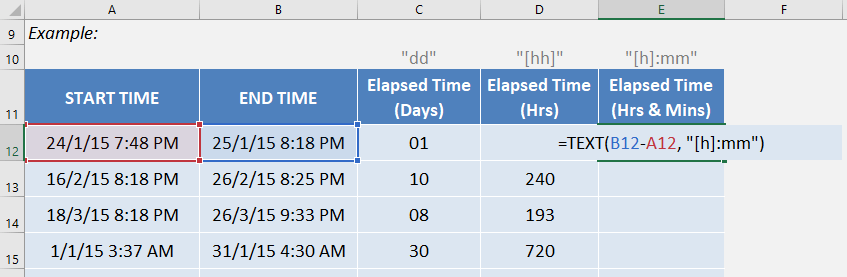STEP 6: Apply the same formula to the rest of the cells by dragging the lower right corner downwards. And your elapsed time results are all ready!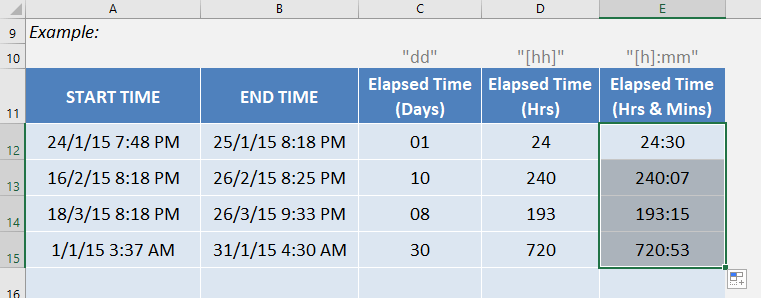How to Use the Text Formula in Excel